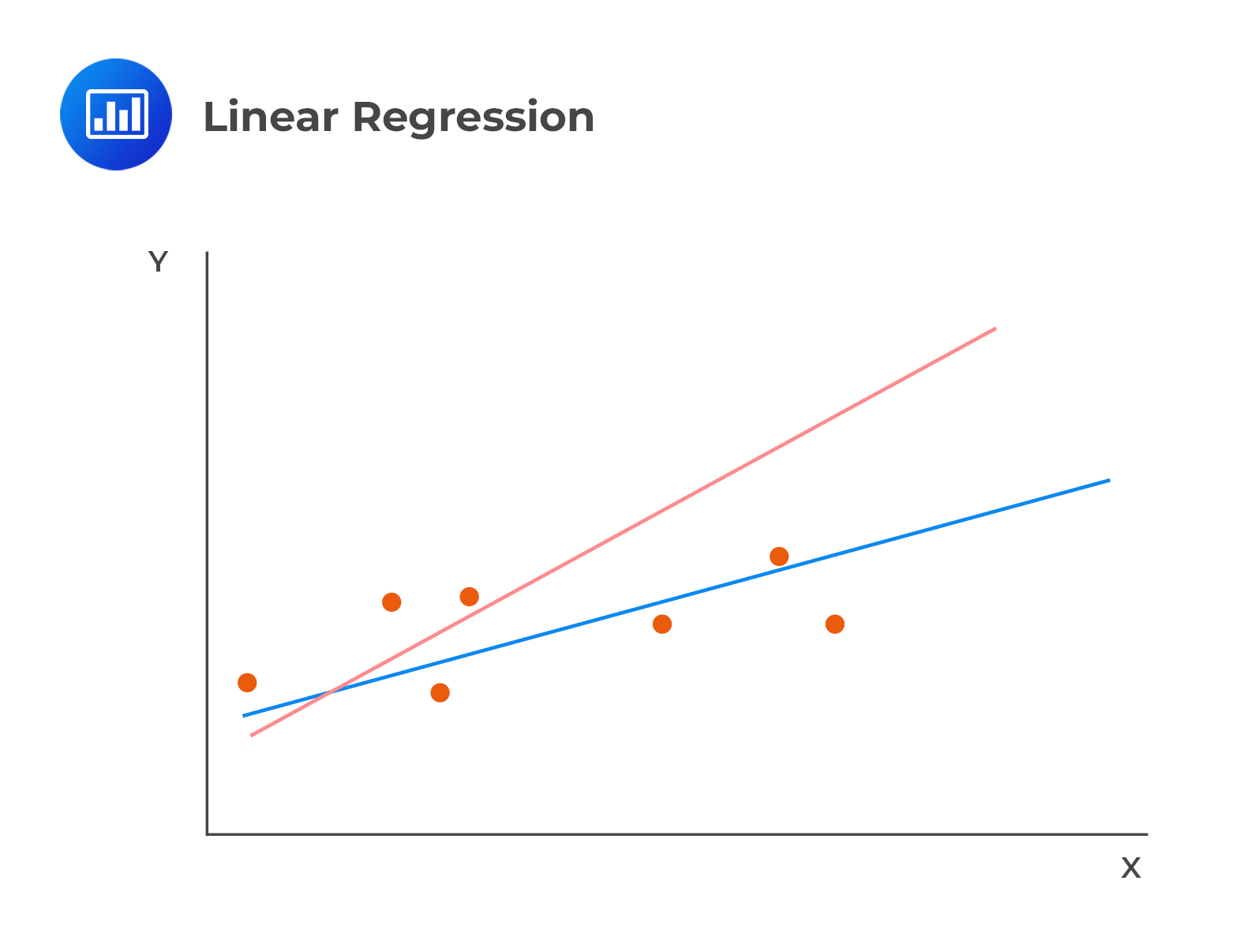Limited Time Offer: Save 10% on all 2022 Premium Study Packages with promo code: BLOG10# The Least Squares Criterion

The linear relation between the dependent and independent variables is described as follows:

$$Y_i =\beta_0+\beta_1X_i+\epsilon_i,\ i=1,2,…,n$$

Where:

$$Y$$ = dependent variable.

$$X$$ = independent variable.

$$\beta_0$$ = intercept.

$$\beta_1$$ = slope coefficient.

$$\epsilon$$ = error term which is the observed value of Y, the expected value of Y.

The intercept, $$\beta_0$$ and the slope coefficient, b1, are known as the regression coefficients.

## Least Squares Criterion

Linear regression calculates a line that best fits the observations. In the following image, the line that best fits the regression is clearly the blue line:The model chooses the estimated or fitted parameters ($$\beta_0$$ and $$\beta_1$$) that minimize the sum of the squared vertical differences between the observations and the regression line (Sum of squared errors (SSE), otherwise referred to as the residual sum of squares). Choosing $$\beta_0$$ and $$\beta_1$$ occasions the minimization of SSR.

$$S S E=\sum_{i=1}^{n}\left(Y_{i}-\hat{\beta}_{0}-\hat{\beta}_{1} X_{i}\right)^{2}=\sum_{i=1}^{n}\left(Y_{i}-\hat{Y}_{i}\right)^{2}=\sum_{i=1}^{n} e_{i}^{2}$$

It is important to remember  that $$\beta_0$$ and $$\beta_1$$ are never observed in a regression model. It is only the estimates, $$\hat{\beta}_0$$ and $$\hat{\beta}_1$$ that can be observed.

## Interpreting Coefficients

### The Slope Coefficient ($$\hat{\beta}_1$$)

The slope coefficient is defined as the change in the dependent variable caused by a one-unit change in the value of the independent variable. For a regression with one independent variable, the slope coefficient is estimated as shown below.

$$\hat{\beta}_{1}=\frac{\operatorname{Cov}(X, Y)}{\operatorname{Var}(X)}=\frac{\frac{\sum_{i=1}^{n}\left(Y_{i}-\bar{Y}\right)\left(X_{i}-\bar{X}\right)}{n-1}}{\frac{\sum_{i=1}^{n}\left(X_{i}-\bar{X}\right)^{2}}{n-1}}=\frac{\sum_{i=1}^{n}\left(Y_{i}-\bar{Y}\right)\left(X_{i}-\bar{X}\right)}{\sum_{i=1}^{n}\left(X_{i}-\bar{X}\right)^{2}}$$

### The Intercept ($$\hat{\beta}_0$$)

The intercept is the estimated value of the dependent variable when the independent variable is zero. The fitted regression line passes through the point equivalent to the means of the dependent and the independent variables in a linear regression model.

The intercept can, as such, be expressed as:

$$\hat{\beta}_0=\hat{Y}-\hat{\beta}_1\hat{X}$$

Where:

$$\hat{Y}$$ = mean of Y.

$$\hat{X}$$ = mean of X.

#### Example: Interpreting Regression Coefficients

Arth Shah is using regression analysis to forecast inflation based on unemployment data from 2011 to 2020. Shah has computed the following values.

• $$Cov(X, Y)$$ = – 0.000130734
• $$Var (X)$$ = 0.000144608
• $$\hat{Y}$$ = 0.0234
• $$\hat{X}$$ = 0.0526

We can calculate and interpret the slope coefficient and the intercept term for the regression analysis as follows:

#### Slope Coefficient

\begin{align} \text{Slope Coefficient}(\hat{\beta}_1) &=\frac{Cov(X, Y)}{(Var (X)}\\ &=\frac{-0.000130734}{0.000144608}=-0.90405\end{align}

#### Intercept Term

\begin{align} \hat{\beta}_0 &=\hat{Y}-\hat{\beta}_1\hat{X}\\&=0.0234-(-0.90405)\times 0.0526 =0.070953\end{align}

#### Interpretation

Given the value of the slope coefficient, (-0.90405$$\sim$$ -0.9), we can say that inflation rates decrease (increase) by 0.90 units whenever unemployment rates increase (decrease) by one unit.

# Question

A regression model with one independent variable requires several assumptions for valid conclusions. Which of the following statements most likely violates those assumptions?

1. The independent variable is random.
2. The error term is distributed normally.
3. There exists a linear relationship between the dependent variable and the independent variable.

Solution

Linear regression assumes that the independent variable, X, is NOT random. This ensures that the model produces the correct estimates of the regression coefficients.

B is incorrect. The assumption that the error term is distributed normally allows us to easily test a particular hypothesis about a linear regression model.

C is incorrect. Essentially, the assumption that the dependent and independent variables have a linear relationship is the key to a valid linear regression. If the parameters of the dependent and independent variables are not linear, then the estimation of that relation can yield invalid results.

Shop CFA® Exam Prep

Offered by AnalystPrepLevel I
Level II
Level III
All Three Levels
Featured Shop FRM® Exam PrepFRM Part I
FRM Part II
FRM Part I & Part II
Learn with Us

Subscribe to our newsletter and keep up with the latest and greatest tips for success
Shop Actuarial Exams PrepExam P (Probability)
Exam FM (Financial Mathematics)
Exams P & FM
Shop GMAT® Exam PrepComplete CourseSergio Torrico
2021-07-23
Excelente para el FRM 2 Escribo esta revisión en español para los hispanohablantes, soy de Bolivia, y utilicé AnalystPrep para dudas y consultas sobre mi preparación para el FRM nivel 2 (lo tomé una sola vez y aprobé muy bien), siempre tuve un soporte claro, directo y rápido, el material sale rápido cuando hay cambios en el temario de GARP, y los ejercicios y exámenes son muy útiles para practicar.diana
2021-07-17
So helpful. I have been using the videos to prepare for the CFA Level II exam. The videos signpost the reading contents, explain the concepts and provide additional context for specific concepts. The fun light-hearted analogies are also a welcome break to some very dry content. I usually watch the videos before going into more in-depth reading and they are a good way to avoid being overwhelmed by the sheer volume of content when you look at the readings.Kriti Dhawan
2021-07-16
A great curriculum provider. James sir explains the concept so well that rather than memorising it, you tend to intuitively understand and absorb them. Thank you ! Grateful I saw this at the right time for my CFA prep.nikhil kumar
2021-06-28
Very well explained and gives a great insight about topics in a very short time. Glad to have found Professor Forjan's lectures.Marwan
2021-06-22
Great support throughout the course by the team, did not feel neglectedBenjamin anonymous
2021-05-10
I loved using AnalystPrep for FRM. QBank is huge, videos are great. Would recommend to a friendDaniel Glyn
2021-03-24
I have finished my FRM1 thanks to AnalystPrep. And now using AnalystPrep for my FRM2 preparation. Professor Forjan is brilliant. He gives such good explanations and analogies. And more than anything makes learning fun. A big thank you to Analystprep and Professor Forjan. 5 stars all the way!michael walshe
2021-03-18
Professor James' videos are excellent for understanding the underlying theories behind financial engineering / financial analysis. The AnalystPrep videos were better than any of the others that I searched through on YouTube for providing a clear explanation of some concepts, such as Portfolio theory, CAPM, and Arbitrage Pricing theory. Watching these cleared up many of the unclarities I had in my head. Highly recommended.